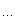const_castreinterpret_caststatic_castdynamic_cast等等。

1）static_cast<T*>(a)class B};class D : public B};void f(B* pb, D* pd){D
* pd2 = static_cast<D*>(pb);        // 不安全, pb可能只是B的指针B
* pb2 = static_cast<B*>(pd);        // 安全的}2）dynamic_cast<T*>(a)class A};class B};void f(){A
* pa = new A;B
* pb = new B;void* pv = dynamic_cast<A*>(pa);// pv 现在指向了一个类型为A的对象pv
= dynamic_cast<B*>(pb);// pv 现在指向了一个类型为B的对象}3）const_cast<T*>(a)class A};void f(){const A *pa = new A;//const对象A
*pb;//非const对象//pb = pa; // 这里将出错，不能将const对象指针赋值给非const对象pb
= const_cast<A*>(pa); // 现在OK了..   }4）reinterpret_cast<T*>(a)class A};class B};void f(){A
* pa = new A;void* pv = reinterpret_cast<A*>(pa);// pv 现在指向了一个类型为B的对象，这可能是不安全的}